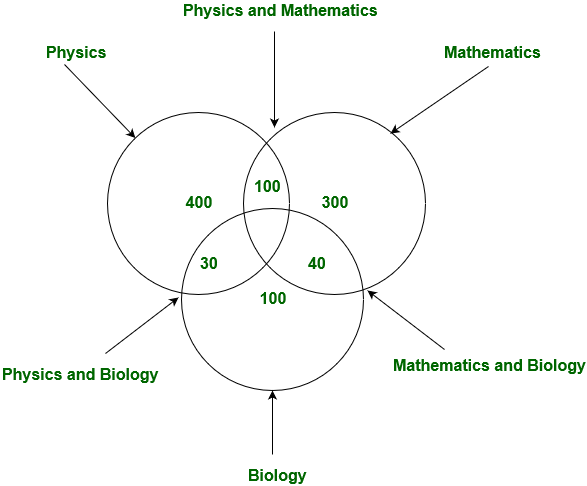GRE Quiz | GRE Quantitative 2 | Question 5

• Last Updated : 14 Mar, 2019

Question 1 – 8 are quantity comparison Questions. Question 5 – 6 are based on the information given below:

In a university there are 1200 students 400 appeared for Physics exam only, 300 students appeared for Mathematics only. 100 students appeared for Biology exam only.100 students appeared for both Physics and Mathematics exam, 30 students appeared for both Physics and Biology test 40 students appeared for Physics and Mathematics. No one appeared for all three exams.

Quantity A: Number of the students not appeared in any exam
Quantity B: Number of the students appeared in more than 1 exam

(A) Quantity A is greater.
(B) Quantity B is greater
(C) The two quantities are equal.
(D) The relationship cannot be determined from the information given.

Explanation:Quantity A: Number of the students not appeared in any exam
= 1200 - [400(Physics) + 300(Mathematics) + 100(Biology)
+ 100(Physics and Mathematics) + 30(Physics and Biology)
+ 40(Physics and Mathematics)]
= 230

Quantity B: Number of the students appeared in more than 1 exam
= 100(Physics and Mathematics) + 30(Physics and Biology)
+ 40(Physics and Mathematics)
= 170

Quantity A is greater than quantity B.

Option (A) is correct.

Quiz of this Question

My Personal Notes arrow_drop_up